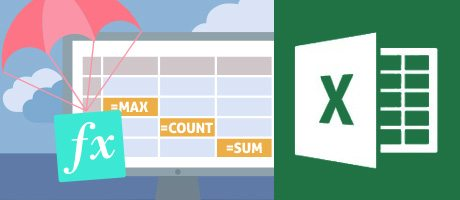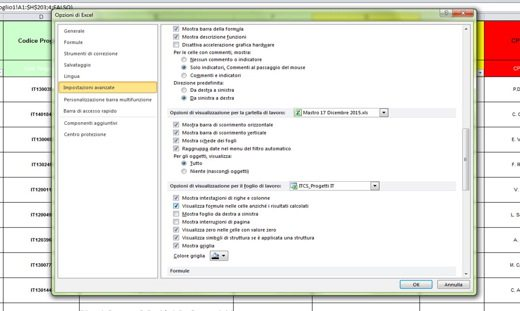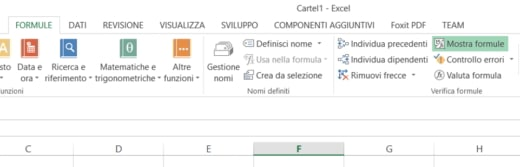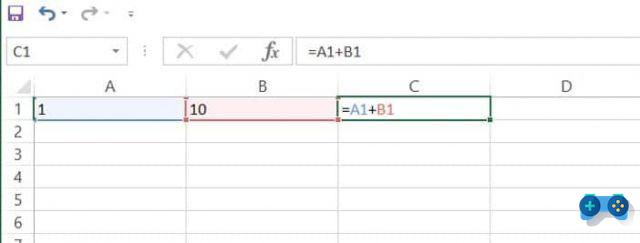# How to view the formulas of an Excel sheetWho I am
Joel Fulleda
@joelfulledaAuthor and references

View the formulas of an Excel sheet it is necessary to have an overview of the calculations made. The formulas that perform the calculations always start with the = sign, followed by mathematical operators, numbers and predefined Excel functions.

If for work you use very large Excel documents, created by other colleagues, and you want to be able to immediately identify the formulas present, continue reading this tutorial because after reading it, you will discover how to view formulas in an excel sheet in a very short time.

Show formulas on an Excel sheet it is very easy and does not require great computer skills. With the advice I will give you, you will understand how to move and above all how to find the information you need to interpret the formulas applied in Excel sheets.The first step in taking a look at the formulas of an Excel sheet is to select the individual cells and take a look at the bar Fx. By clicking on the bar Fx you will be able to view formulas also insert new ones or modify them if necessary.Excel: the formula entered does not return any results

## How to view the formulas of an Excel sheet

For a more detailed analysis on the use of formulas, sifting through the Excel settings, you need to go to the menu Formulas and, in section Check formulas, click on the item Show formulas.

If you want to see which cells contribute to the calculation of the formulas found, positioned on one of them, and, in the same section as before, click on the top menu on Individual previous.Finally, if you are interested in knowing if a cell contributes to the calculation of some formula, click on it and then on Individual employees,it. To eliminate the arrows introduced on the Excel sheet by the previous options, click on the item Remove arrows.

## How to display formulas in cells

You can also go to to view formulas in Excel sheet cells File> Options> Advanced Settings. Here locate the item Display options for the worksheet and check the item Display formulas in cells instead of calculated results.Press on Ok and returning to the Excel spreadsheet you will notice the formulas applied in the cells.To activate this feature with the keyboard shortcut, press simultaneously CTRL+SHIFT+8, and that's it.

## How to delete a formula

If by viewing the identified formulas you want to delete some of them, you can decide whether to remove their results or just the formula.

To delete the formulas along with the resulting values ​​you need to select the cell or range of cells that contains the formula and press the key on the keyboard DEL.

To delete formulas without removing the resulting values ​​you need to select the cell or range of cells that contains the formula and in the group Clipboard of the card Home, click on Copy and then in the group Clipboard of the card Home, click on Paste> Paste Values.

### Related Items

##### add a comment of How to view the formulas of an Excel sheet
Comment sent successfully! We will review it in the next few hours.